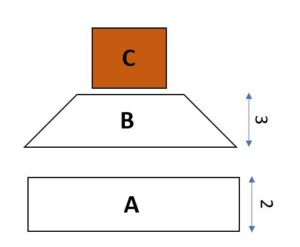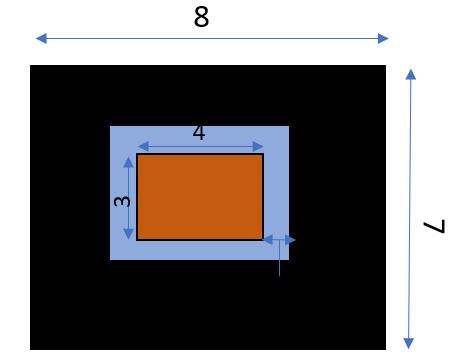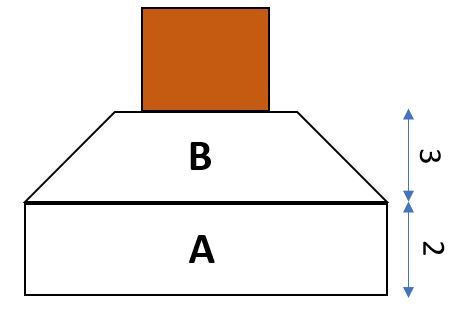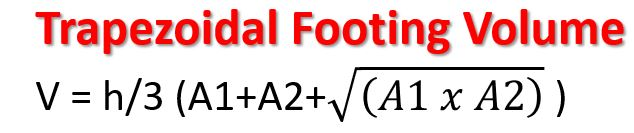# Trapezoidal Footing Formula with Example

## Trapezoidal Footing Concrete Formula

### Trapezoidal Footing

1. Rectangular Cubes (A)
2. Truncated Pyramid Cubes  (B)

### Please See the Image Below:If you want to calculate whole shape volume, you have to calculate both shapes one by one.

Let us Assume the whole shape size and dimensions.

Column Size- 3m X 4m

Column to Top edge Spacing- 0.5m

Whole Footing Size -7m X 8m

The total height of footing is 6m

A Shape Height is 2m

B shape Height is 3mNow, we have the whole structure data, Now time to put all data in Formula.

First, we calculate Shape A

as you can see shape A is a just rectangular shape

we know the whole size of the footing is 7m X8m and the A shape Height is 2 m.

Area of A shape

A1 = L x W

A1= 8 x 7 = 56 m2

The volume of A Shape

Volume = Area x height

Volume = 56 x 2 = 112 m3 ———-A

### Now we are going to calculate the Truncated Pyramid Cubes’ shapeV = h/3 (A1+A2+√((A1 x A2) ) )

Where h is the height of the trapezoidal shape

A1 Area of the lower shape

A2 Area of upper shape

A1 = L x W                        A2 = L x W

A1 = 7 x 8                        A2 = 5 x 4

A1 = 56 m                   A2 = 20 m2

V =3/3 (56+20+√((56 x 20) ) )

V =109.46 m3 ————B

Above we calculate the volume of A (112 m3)

Now we add A+B= 221.46 m3

Trapezoidal Footing Total Volume is 221.46 m3.

For a Better Experience, you can watch this video.Channel for more information.
1.Ajay Kumar says: